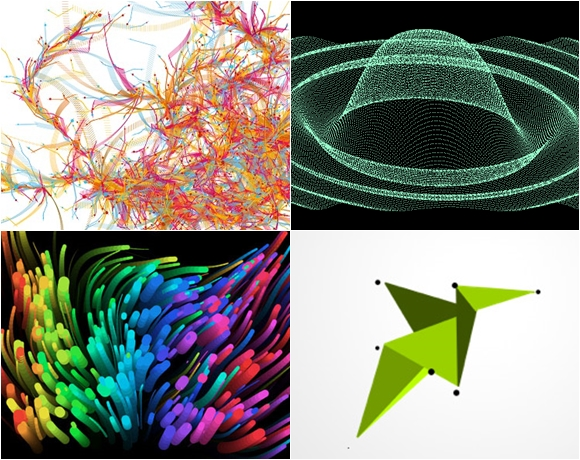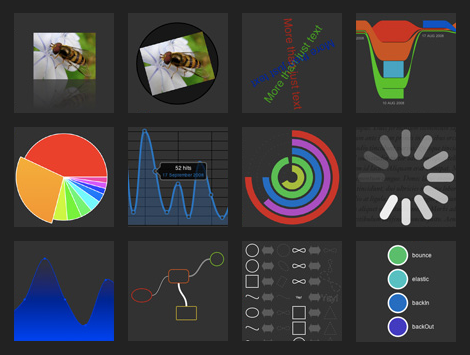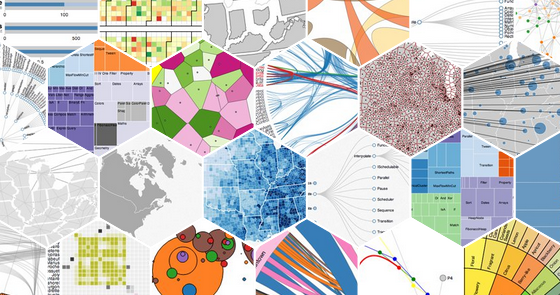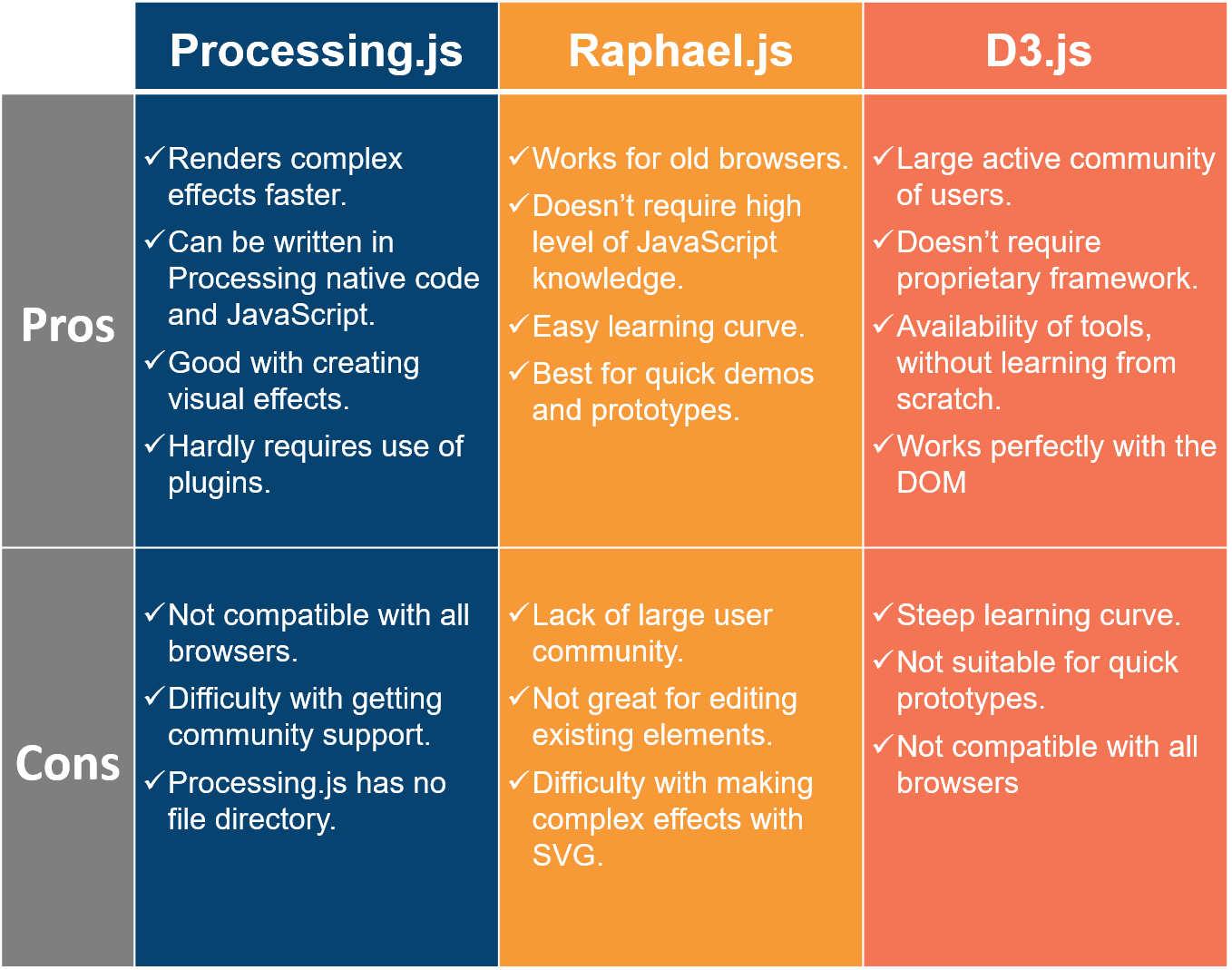{{announcement.body}}
{{announcement.title}}

# JavaScript Visualization Frameworks Review

DZone 's Guide to

# JavaScript Visualization Frameworks Review

### If you're a JavaScript developer who's interested in working with data, but you don't have time to learn Python or R, check out these great frameworks!

· Web Dev Zone ·
Free Resource

Comment (0)

Save
{{ articles.views | formatCount}} Views

Note: If you're interested in seeing the cool results of the code in this article, try plugging them into jsfiddle.net.

Data is driving the world, and at this stage when the world is being held ransom by data and the web, it only makes sense to read information into the lots of data being generated out there.

A language like JavaScript is very important in the manner in which a web page gets to be displayed, and it makes complete sense for it to have a means of displaying data to the client.

Fortunately for the JavaScript evangelists, frontend developers, and data scientists, this article discusses three major JavaScript frameworks for visualizing data to the client without having to switch to a language which has been touted to be more data related such as Python and R.

The three major drawing tools that this article covers include Processing.js, Raphael.js, and D3.js.

## Processing.jsProcessing is both a language and programming environment, with a Java-like syntax as its native code, originally developed by Ben Fry and Casey Reas in 2001.

It was then ported to JavaScript by John Resig to be open sourced in 2008.

Users of Processing call their applications sketches, and Processing.js simply converts the native code into JavaScript in order to render them on a web page.

``````<html>
<meta charset="UTF-8">
<title>Hello World!</title>
<body>
<script src="https://rawgit.com/processing-js/processing-js/v1.4.8/processing.js"></script>
<script type="text/processing" data-processing-target="mycanvas">
boolean autoplay = false;
boolean showsFPS = false;
boolean clearsBackground = true;
boolean windEnabled = true;
PFont font;
float rotRange = 10;
float rotDecay = 1.1;
float sizeDecay = 0.7;
float lengthDecay = 0.91;
int levelMax = 8;
int leafLevel = 2;
float leafChance = 0.3;
float branchHue = 50;
float leafHue = 150;
float leafSat = 100;
float mouseWind = 0;
float mouseWindV = 0;
float startLength;
float startSize;
color trunkColor;
color bgColor;
int time = 0;
float lengthRand = 1.0;
float bloomWidthRatio = 0.6;
float bloomSizeAverage = 15;

float mDamp = 0.00002;
float wDamp = 0.003;
float mFriction = 0.98;

float flowerChance = 0.1;
color flowerColor;
float flowerWidth = 10;
float flowerHeight = 20;

Node node;

void setup()
{
size(940, 540);
colorMode(HSB);
font = createFont("Helvetica", 24);
ellipseMode(CENTER);

randomize();
reset();
}

void draw()
{
if (autoplay)
{
time++;
if (time > 600)
{
time = 0;
randomize();
reset();
}
}

float dx = mouseX - pmouseX;
mouseWindV += dx * mDamp;
mouseWindV += (0 - mouseWind) * wDamp;
mouseWindV *= mFriction;
mouseWind += mouseWindV;

if (clearsBackground) background(bgColor);
if (showsFPS) displayFPS();
translate(width/2, height);
node.draw();
}

void reset()
{
background(bgColor);
node = new Node(startLength, startSize, rotRange, 0);
}

void randomize()
{
randomizeBackground();
randomizeColor();
rotRange = random(20, 60);
rotDecay = random(0.9, 1.1);
startLength = random(20, 80);
startSize = random(3, 20);
lengthRand = random(0.0, 0.2);
leafChance = random(0.3, 0.9);
sizeDecay = random(0.6, 0.7);
lengthDecay = map(startLength, 20, 80, 1.1, 0.85);
leafLevel = random(0, 4);
bloomWidthRatio = random(0.01, 0.9);
bloomSizeAverage = random(10, 40);

mDamp = 0.00002;
wDamp = 0.005;
mFriction = 0.96;

flowerWidth = random(5, 15);
flowerHeight = random(10, 30);
flowerChance = 0.1;
}

void randomizeBackground()
{
bgColor = color(random(255), random(0, 100), 255);
}

void randomizeColor()
{
branchHue = random(0, 255);
leafHue = random(0, 255);
leafSat = random(0, 255);
flowerColor = color(random(255), random(0, 255), 255);
if (node) node.randomizeColor();
}

void displayFPS()
{
textFont(font, 18);
fill(150);
String output = "fps=";
output += (int) frameRate;
text(output, 10, 30);
}

void keyPressed()
{
if (key == 'f') showsFPS = !showsFPS;
if (key == 'a') autoplay = !autoplay;
if (key == 'p') clearsBackground = !clearsBackground;
if (key == 'w') windEnabled = !windEnabled;
if (key == 'r') reset();
if (key == 'b') randomizeBackground();
if (key == 'c') randomizeColor();
}

void mousePressed()
{
time = 0;
randomize();
reset();
}
class Node
{
float len;
float size;
float rot;
int level;
float s = 0;
float windFactor = 1.0;
boolean doesBloom;
color branchColor;
float bloomSize;
color leafColor;
float leafRot;
float leafScale = 0.0;
int leafDelay;
boolean doesFlower;
float flowerScale = 0.0;
float flowerScaleT = 1.0;
float flowerBright = 255;
int flowerDelay;

Node n1;
Node n2;

Node(float _len, float _size, float _rotRange, int _level)
{
len = _len * (1 + random(-lengthRand, lengthRand));
size = _size;
level = _level;
if (level < leafLevel) rot *= 0.3;
if (level == 0 ) rot = 0;
windFactor = random(0.2, 1);
doesBloom = false;
if (level >= leafLevel && random(1) < leafChance) doesBloom = true;
bloomSize = random(bloomSizeAverage*0.7, bloomSizeAverage*1.3);
flowerScaleT = random(0.8, 1.2);
flowerDelay = round(random(200, 250));
leafDelay = round(random(50, 150));
randomizeColor();

if (random(1) < flowerChance) doesFlower = true;

float rr = _rotRange * rotDecay;

if (level < levelMax)
{
n1 = new Node(len*lengthDecay, size*sizeDecay, rr, level+1);
n2 = new Node(len*lengthDecay, size*sizeDecay, rr, level+1);
}
}

void draw()
{
strokeWeight(size);
s += (1.0 - s) / (15 + (level*5));
scale(s);

pushMatrix();

if (level >= leafLevel) stroke(branchColor);
else stroke(0);
float rotOffset = sin( noise( (float)millis() * 0.000006  * (level*1) ) * 100 );
if (!windEnabled) rotOffset = 0;
rotate(rot + (rotOffset * 0.1 + mouseWind) * windFactor);
line(0, 0, 0, -len);
translate(0, -len);

// draw leaves
if (doesBloom)
{
if (leafDelay < 0)
{
leafScale += (1.0 - leafScale) * 0.05;
fill(leafColor);
noStroke();
pushMatrix();
scale(leafScale);
rotate(leafRot);
translate(0, -bloomSize/2);
ellipse(0, 0, bloomSize*bloomWidthRatio, bloomSize);
popMatrix();
}
else
{
leafDelay--;
}
}

// draw flowers
if (doesFlower && level > levelMax-3)
{
if (flowerDelay < 0)
{
pushMatrix();
flowerScale += (flowerScaleT - flowerScale) * 0.1;
scale(flowerScale);
rotate(flowerScale*3);
noStroke();
fill(hue(flowerColor), saturation(flowerColor), flowerBright);
ellipse(0, 0, flowerWidth, flowerHeight);
ellipse(0, 0, flowerWidth, flowerHeight);
ellipse(0, 0, flowerWidth, flowerHeight);
fill(branchColor);
ellipse(0, 0, 5, 5);
popMatrix();
} else
{
flowerDelay--;
}
}

pushMatrix();
if (n1) n1.draw();
popMatrix();

pushMatrix();
if (n2) n2.draw();
popMatrix();

popMatrix();
}

void randomizeColor()
{
branchColor = color(branchHue, random(170, 255), random(100, 200));
leafColor = color(leafHue, leafSat, random(100, 255));
flowerBright = random(200, 255);

if (n1) n1.randomizeColor();
if (n2) n2.randomizeColor();
}
}
</script>
<canvas id="mycanvas"></canvas>
<!--        <canvas data-processing-sources="infinite-arboretum.js"></canvas> -->
</body>
</html>``````

It also has setup() and draw() functions, that are used to initialize the application state and draw on the canvas element, respectively.

The process of learning to use the Processing.js framework is beyond the scope of this article. However, the online reference can be found here.

It is encouraging to know that:

• It also allows for user interaction, as it can take input from them; thereby making it a very resourceful tool.

Processing.js can be downloaded here. By the time you get to master or work with it, you can contribute to the project.

As powerful as this tool is, it is said to be the least powerful of the three frameworks to be discussed in today’s article.

That takes us to the next framework.

## Raphael.jsRaphael’s strongest point is said to be its ability to allow for easy drawing. To make use of Raphael, all you need is a browser and text editor. However; that’s not the most exciting part.

Raphael also:

• Allows for coding. You can write commands for it to draw things in a unique way when a user loads your web page.

• Supports the <canvas> element in HTML5.

• Works on almost all browsers.

• Doesn’t require external plugins to function properly.

• It's mobile device friendly.

Raphael works directly with browser’s built-in graphics language known as Scalable Vector Graphics (SVG).

#### JS Inserted in HTML

``````<html lang="en"><head><meta http-equiv="Content-Type" content="text/html; charset=UTF-8">

<script src="https://rawgit.com/DmitryBaranovskiy/raphael/master/raphael.min.js" type="text/javascript" charset="utf-8"></script>
<script type="text/javascript" charset="utf-8">
var r = Raphael("holder", 640, 480),
angle = 0;
while (angle < 360) {
var color = Raphael.getColor();
(function (t, c) {
r.circle(320, 450, 20).attr({stroke: c, fill: c, transform: t, "fill-opacity": .4}).click(function () {
s.animate({transform: t, stroke: c}, 2000, "bounce");
}).mouseover(function () {
this.animate({"fill-opacity": .75}, 500);
}).mouseout(function () {
this.animate({"fill-opacity": .4}, 500);
});
})("r" + angle + " 320 240", color);
angle += 30;
}
Raphael.getColor.reset();
var s = r.set();
s.push(r.path("M320,240c-50,100,50,110,0,190").attr({fill: "none", "stroke-width": 2}));
s.push(r.circle(320, 450, 20).attr({fill: "none", "stroke-width": 2}));
s.push(r.circle(320, 240, 5).attr({fill: "none", "stroke-width": 10}));
s.attr({stroke: Raphael.getColor()});
};
</script>
<body>
<div id="holder"><svg height="480" version="1.1" width="640" xmlns="http://www.w3.org/2000/svg" style="overflow: hidden; position: relative; top: -0.5px;"><desc style="-webkit-tap-highlight-color: rgba(0, 0, 0, 0);">Created with Raphaël 2.0.0</desc><defs style="-webkit-tap-highlight-color: rgba(0, 0, 0, 0);"></defs><circle cx="320" cy="450" r="20" fill="#bf0000" stroke="#bf0000" transform="matrix(1,0,0,1,0,0)" fill-opacity="0.4" style="-webkit-tap-highlight-color: rgba(0, 0, 0, 0); fill-opacity: 0.4;"></circle><circle cx="320" cy="450" r="20" fill="#bf5600" stroke="#bf5600" transform="matrix(0.866,0.5,-0.5,0.866,162.8719,-127.8461)" fill-opacity="0.4" style="-webkit-tap-highlight-color: rgba(0, 0, 0, 0); fill-opacity: 0.4;"></circle><circle cx="320" cy="450" r="20" fill="#bfac00" stroke="#bfac00" transform="matrix(0.5,0.866,-0.866,0.5,367.8461,-157.1281)" fill-opacity="0.4" style="-webkit-tap-highlight-color: rgba(0, 0, 0, 0); fill-opacity: 0.4;"></circle><circle cx="320" cy="450" r="20" fill="#7cbf00" stroke="#7cbf00" transform="matrix(0,1,-1,0,560,-80)" fill-opacity="0.4" style="-webkit-tap-highlight-color: rgba(0, 0, 0, 0); fill-opacity: 0.4;"></circle><circle cx="320" cy="450" r="20" fill="#26bf00" stroke="#26bf00" transform="matrix(-0.5,0.866,-0.866,-0.5,687.8461,82.8719)" fill-opacity="0.4" style="-webkit-tap-highlight-color: rgba(0, 0, 0, 0); fill-opacity: 0.4;"></circle><circle cx="320" cy="450" r="20" fill="#00bf2f" stroke="#00bf2f" transform="matrix(-0.866,0.5,-0.5,-0.866,717.1281,287.8461)" fill-opacity="0.4" style="-webkit-tap-highlight-color: rgba(0, 0, 0, 0); fill-opacity: 0.4;"></circle><circle cx="320" cy="450" r="20" fill="#00bf85" stroke="#00bf85" transform="matrix(-1,0,0,-1,640,480)" fill-opacity="0.4" style="-webkit-tap-highlight-color: rgba(0, 0, 0, 0); fill-opacity: 0.4;"></circle><circle cx="320" cy="450" r="20" fill="#00a2bf" stroke="#00a2bf" transform="matrix(-0.866,-0.5,0.5,-0.866,477.1281,607.8461)" fill-opacity="0.4" style="-webkit-tap-highlight-color: rgba(0, 0, 0, 0); fill-opacity: 0.4;"></circle><circle cx="320" cy="450" r="20" fill="#004cbf" stroke="#004cbf" transform="matrix(-0.5,-0.866,0.866,-0.5,272.1539,637.1281)" fill-opacity="0.4" style="-webkit-tap-highlight-color: rgba(0, 0, 0, 0); fill-opacity: 0.4;"></circle><circle cx="320" cy="450" r="20" fill="#0900bf" stroke="#0900bf" transform="matrix(0,-1,1,0,80,560)" fill-opacity="0.4" style="-webkit-tap-highlight-color: rgba(0, 0, 0, 0); fill-opacity: 0.4;"></circle><circle cx="320" cy="450" r="20" fill="#5f00bf" stroke="#5f00bf" transform="matrix(0.5,-0.866,0.866,0.5,-47.8461,397.1281)" fill-opacity="0.4" style="-webkit-tap-highlight-color: rgba(0, 0, 0, 0); fill-opacity: 0.4;"></circle><circle cx="320" cy="450" r="20" fill="#b500bf" stroke="#b500bf" transform="matrix(0.866,-0.5,0.5,0.866,-77.1281,192.1539)" fill-opacity="0.4" style="-webkit-tap-highlight-color: rgba(0, 0, 0, 0); fill-opacity: 0.4;"></circle><path fill="none" stroke="#0900bf" d="M320,240C270,340,370,350,320,430" stroke-width="2" style="-webkit-tap-highlight-color: rgba(0, 0, 0, 0);" transform="matrix(0,-1,1,0,80,560)"></path><circle cx="320" cy="450" r="20" fill="none" stroke="#0900bf" stroke-width="2" style="-webkit-tap-highlight-color: rgba(0, 0, 0, 0);" transform="matrix(0,-1,1,0,80,560)"></circle><circle cx="320" cy="240" r="5" fill="none" stroke="#0900bf" stroke-width="10" style="-webkit-tap-highlight-color: rgba(0, 0, 0, 0);" transform="matrix(0,-1,1,0,80,560)"></circle></svg></div>
<p id="copy">Demo of <a href="http://raphaeljs.com/">Raphaël</a>—JavaScript Vector Library</p>

</body></html>``````

#### CSS

``````body {
background: #333;
color: #fff;
font: 300 100.1% "Helvetica Neue", Helvetica, "Arial Unicode MS", Arial, sans-serif;
}
#holder {
height: 480px;
left: 50%;
margin: -240px 0 0 -320px;
position: absolute;
top: 50%;
width: 640px;
}
#copy {
bottom: 0;
font: 300 .7em "Helvetica Neue", Helvetica, "Arial Unicode MS", Arial, sans-serif;
position: absolute;
right: 1em;
text-align: right;
}
#copy a {
color: #fff;
}

#holder svg:nth-child(2) {
display: none;
}``````

However, the main reason Raphael is compatible with older browsers is that it works well with a format known as Vector Markup Language (VML).

Unlike Processing.js, which had to be ported from Processing and is a Java-based language, Raphael is purely JavaScript; meaning it works seamlessly and naturally with web pages. Raphael.js is a more popular framework than Processing.js. It also has lots of users in the data visualization community and you would have people to turn to whenever anything goes wrong.

It is open source and will definitely be improved upon. All these features give Raphael.js an edge over a lot of the other data visualization frameworks out there.

One more thing is that Raphael has an easy learning curve and can be used almost immediately.

That takes us to the next one which is said to be the best framework for visualization but has a steep learning curve.

## D3.jsD3 stands for (Data-Driven Documents) and, as the name implies, it is well packaged for data visualization purposes. It translates raw datasets into visualizations and works seamlessly with Microsoft Excel. D3 was created by one of the leading evangelists of web data visualization, Mike Bostock.

Just like Raphael.js, D3 works with SVG and has the visualization shapes as part of the DOM (Document Object Model). Meaning you can style and design your data, making use of Cascading Style Sheets (CSS).

#### JS Inserted in HTML

``````Collision Detection<meta charset="utf-8">
<body>
<script src="//d3js.org/d3.v3.min.js"></script>
<script>

var width = 960,
height = 500;

var nodes = d3.range(200).map(function() { return {radius: Math.random() * 12 + 4}; }),
root = nodes,
color = d3.scale.category10();

root.fixed = true;

var force = d3.layout.force()
.gravity(0.05)
.charge(function(d, i) { return i ? 0 : -2000; })
.nodes(nodes)
.size([width, height]);

force.start();

var svg = d3.select("body").append("svg")
.attr("width", width)
.attr("height", height);

svg.selectAll("circle")
.data(nodes.slice(1))
.enter().append("circle")
.attr("r", function(d) { return d.radius; })
.style("fill", function(d, i) { return color(i % 3); });

force.on("tick", function(e) {
i = 0,
n = nodes.length;

while (++i < n) q.visit(collide(nodes[i]));

svg.selectAll("circle")
.attr("cx", function(d) { return d.x; })
.attr("cy", function(d) { return d.y; });
});

svg.on("mousemove", function() {
var p1 = d3.mouse(this);
root.px = p1;
root.py = p1;
force.resume();
});

function collide(node) {
var r = node.radius + 16,
nx1 = node.x - r,
nx2 = node.x + r,
ny1 = node.y - r,
ny2 = node.y + r;
return function(quad, x1, y1, x2, y2) {
var x = node.x - quad.point.x,
l = Math.sqrt(x * x + y * y),
if (l < r) {
l = (l - r) / l * .5;
node.x -= x *= l;
node.y -= y *= l;
}
}
return x1 > nx2 || x2 < nx1 || y1 > ny2 || y2 < ny1;
};
}

</script>

D3.js is based on HTML, DOM, CSS, SVG and also supports Canvas. D3 has lots of other frameworks built on it that allow for drag and drop. Due to its steep learning curve, D3 is a great long-term investment type of tool, as it allows you to create your own libraries, that you can then use to speed up the creation process.

For data visualization purposes, the most important interaction with SVG is to bind data to around its files, and D3 allows for the rewriting of the data wrapper around SVG files. As it stands, D3.js is not just better than Raphael.js and Processing.js in many cases, but is also a viable replacement for jQuery and other frameworks. However, D3 is said to have a steep learning curve and is most conducive to programmers with experience using JavaScript.

Tools built on D3 include MetricsGraphics, Epoch, Vega, NVD3, and many more. These tools can be used instead of learning D3 from scratch. To get started with D3, head to the D3 website and download the latest version.

## Wrapping Up

Still not sure which framework to choose?

When deciding what framework to use for your data visualization projects, you have to know your priorities. Hopefully, the following pros and cons would help you define them:Topics:
processing js ,d3.js ,raphael.js ,data visualization ,web dev

Comment (0)

Save
{{ articles.views | formatCount}} Views

Published at DZone with permission of Anton Shaleynikov . See the original article here.

Opinions expressed by DZone contributors are their own.